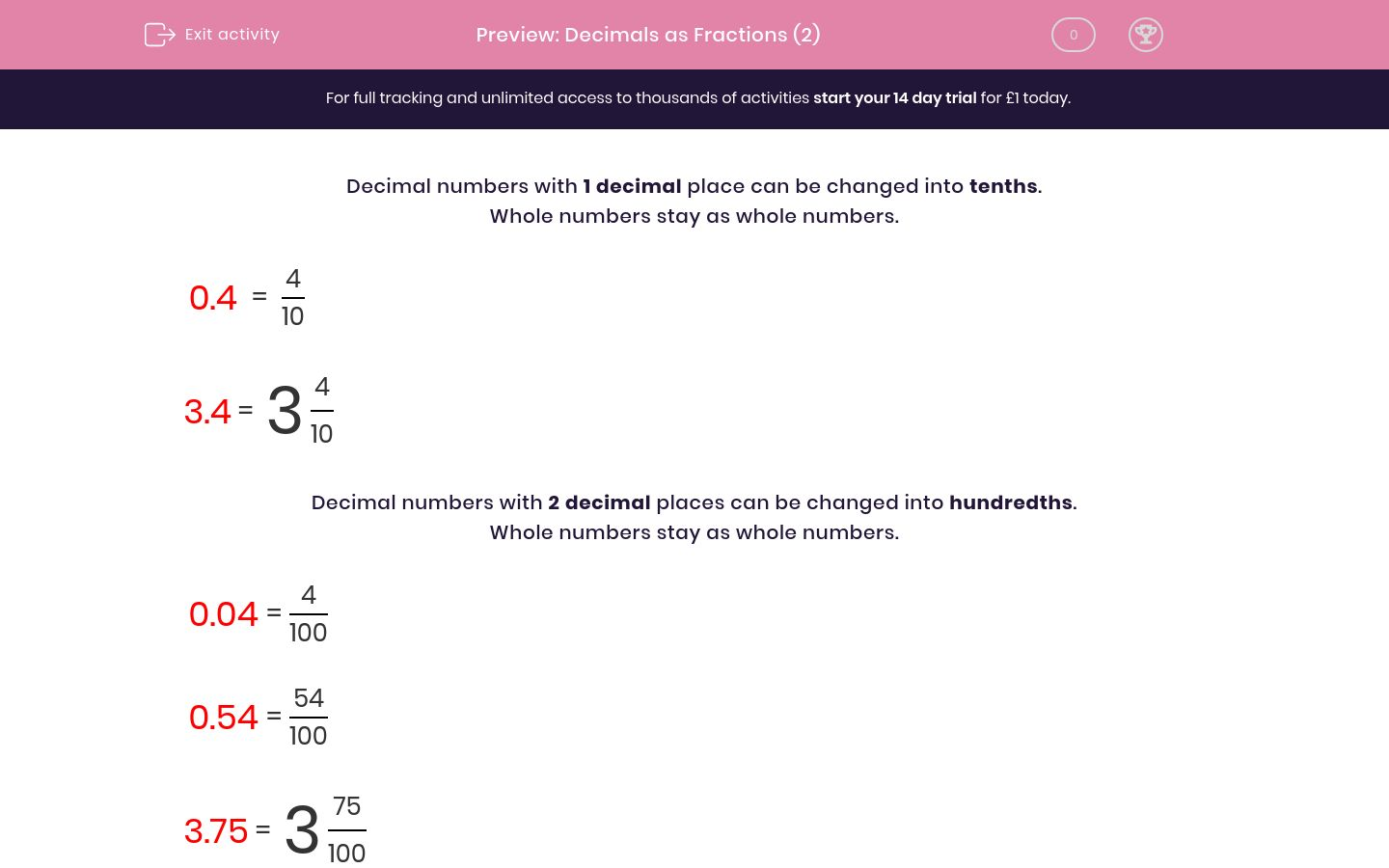# Decimals as Fractions (2)

In this worksheet, students must read and write decimal numbers as fractions or mixed numbers.Key stage:  KS 2

Curriculum topic:   Maths and Numerical Reasoning

Curriculum subtopic:   Decimals

Difficulty level:### QUESTION 1 of 10

Decimal numbers with 1 decimal place can be changed into tenths.

Whole numbers stay as whole numbers.

 0.4 = 4 10

 3.4 = 3 4 10

Decimal numbers with 2 decimal places can be changed into hundredths.

Whole numbers stay as whole numbers.

 0.04 = 4 100

 0.54 = 54 100

 3.75 = 3 75 100

Is this true or false?

 0.08 = 8 100
true

false

Is this true or false?

 0.6 = 6 100
true

false

Is this true or false?

 3.5 = 3 5 10
true

false

Is this true or false?

 3.05 = 3 5 100
true

false

Is this true or false?

 4.17 = 4 17 10
true

false

Is this true or false?

 5.3 = 3 5 10
true

false

Is this true or false?

 5.0 = 5
true

false

Is this true or false?

 9.99 = 9 99 100
true

false

Is this true or false?

 6.87 = 6 87 10
true

false

Is this true or false?

 12.8 = 8 12 100
true

false

• Question 1

Is this true or false?

 0.08 = 8 100
true
EDDIE SAYS
2 decimal places so 8 hundredths.
• Question 2

Is this true or false?

 0.6 = 6 100
false
EDDIE SAYS
1 decimal place so 6 tenths.
• Question 3

Is this true or false?

 3.5 = 3 5 10
true
EDDIE SAYS
1 decimal place so 5 tenths.
The whole number stays as 3.
• Question 4

Is this true or false?

 3.05 = 3 5 100
true
EDDIE SAYS
2 decimal places so 5 hundredths.
The whole number stays as 3.
• Question 5

Is this true or false?

 4.17 = 4 17 10
false
EDDIE SAYS
2 decimal places so 17 hundredths.
The whole number stays as 4.
• Question 6

Is this true or false?

 5.3 = 3 5 10
false
EDDIE SAYS
1 decimal place so 3 tenths.
The whole number stays as 5.
• Question 7

Is this true or false?

 5.0 = 5
true
EDDIE SAYS
1 decimal place so 0 tenths, which we can leave out.
The whole number stays as 5.
• Question 8

Is this true or false?

 9.99 = 9 99 100
true
EDDIE SAYS
2 decimal places so 99 hundredths.
The whole number stays as 9.
• Question 9

Is this true or false?

 6.87 = 6 87 10
false
EDDIE SAYS
2 decimal places so 87 hundredths.
The whole number stays as 6.
• Question 10

Is this true or false?

 12.8 = 8 12 100
false
EDDIE SAYS
1 decimal place so 8 tenths.
The whole number stays as 12.
---- OR ----